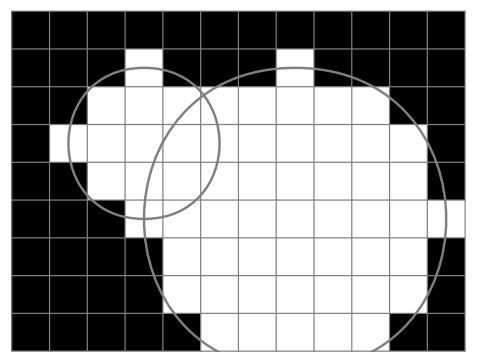시간 제한 메모리 제한 제출 정답 맞은 사람 정답 비율
3 초 256 MB 0 0 0 0.000%

## 문제

Yesterday Andrew wrote a program that draws $n$ white circles on a black screen. The screen is monochrome and it has a resolution $w \times h$ pixels. Pixels are numbered from upper left corner $(0, 0)$ to bottom right one $(w-1, h-1)$.

A circle with the center at pixel $(x_c, y_c)$ and the radius $r$ consists of the pixels with coordinates $(x, y)$ such that $\sqrt{(x_c - x)^2 + (y_c - y)^2} \le r$. If the circle does not fit on the screen, it is truncated. If some pixel belongs to two or more circles, it is white.The resulting picture was very nice, so Andrew decided to copy it to his wall. He has white wallpaper and he can only draw some parts of wall into black. Now he wants to know the amount of paint he needs. He copies the picture exactly pixel-to-pixel, so you should write a program that calculates the number of black pixels left on a screen after drawing $n$ circles.

## 입력

In the first line of input file there are three integers: $w$, $h$, and $n$ ($1 \le w, h \le 20\,000$; $1 \le n \le 100$). Each of the following $n$ lines contains descriptions of the circle. In $i+1$-th line there are three integers: $x_i$, $y_i$, $r_i$ ($0 \le x_i < w$; $0 \le y_i < h$; $0 \le r_i \le 40\,000$). They denote a circle with the center at pixel $(x_i, y_i)$ and radius $r_i$.

## 출력

You should output exactly one number --- the number of black pixels left on the screen.

## 예제 입력 1

5 3 2
1 1 1
3 1 1


## 예제 출력 1

6


## 예제 입력 2

12 9 2
3 3 2
7 5 4


## 예제 출력 2

51


## 노트

The picture corresponds to the second example.